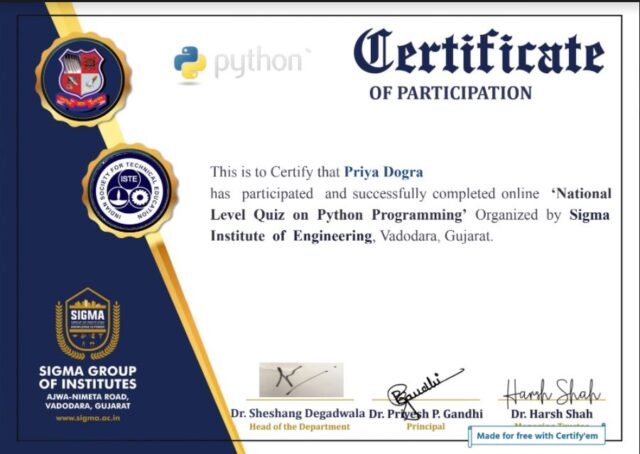Home Certification National Level Python Programming Certificate | Sigma Institute Certificate

# National Level Python Programming Certificate | Sigma Institute Certificate

0This Python Test is designed to help you assess your knowledge of basic Python.This is also a useful resource for employers to examine the technical knowledge of the candidates during an interview or for applicants who want a Python practice test.If you pass this test with 80% or above (16 questions or more), it is likely that you have pretty solid fundamental knowledge of Python!

Join my Whatsapp Group

What will be the output of below Python code? tupl=() tupl1=tupl*2 print(len(tupl1)) *

Error as tuple object has no attribute to len
0
1
2

What will be the output of below Python code? tuple1=(2,4,3) tuple3=tuple1*2 print(tuple3) *

(4,8,6)
Error
(2,4,3,2,4,3)
(2,2,4,4,3,3)

What will be the output of below Python code?list1=[8,0,9,5]print(list1[::-1]) *

[5,9,0,8]
[8,0,9]
[8,0,9,5]
[0,9,5]

CMSB (Council for Media and Satellite Broadcasting)Government Certificate

What will be the result after the execution of above Python code?list1=[3,2,5,7,3,6]list1.pop(3)print(list1) *

[3,2,5,3,6]
[2,5,7,3,6]
[2,5,7,6]
[3,2,5,7,3,6]

What will be the output of following Python code?set1={2,5,3}set2={3,1}set3={}set3=set1&set2print(set3) *

{3}
{}
{2,5,3,1}
{2,5,1}

What will be the output of below Python code?str1=”Information”print(str1[2:8]) *

format
formatio
orma
ormat

Which of the following will result in an error?str1=”python” *

print(str1)
str1=”x”
print(str1[0:9])
Both (b) and (c)

Which of the following is correct with respect to above Python code?d={“a”:3,”b”:7} *

a dictionary d is created.
a and b are the keys of dictionary d.
3 and 7 are the values of dictionary d
All of the above.

What will be the output of the following Python code snippet? print(‘ab cd ef’.title()) *

Ab cd ef
Ab cd eF
Ab Cd Ef
None of the mentioned

In data science, which of the python library are more popular ? *

Numpy
Pandas
OpenCv
Django

What is the output when we execute list(“hello”)? *

[‘hello’]
[‘llo’]
[‘olleh’]
[‘h’, ‘e’, ‘l’, ‘l’, ‘o’]

What will be the output of the following Python code?>>>t = (1, 2)>>>2 * t *

(1, 1, 2, 2)
[1, 1, 2, 2]
(1, 2, 1, 2)
[1, 2, 1, 2]

Which of the following is an invalid statement? *

abc = 1,000,000
a b c = 1000 2000 3000
a,b,c = 1000, 2000, 3000
a_b_c = 1,000,000

What is the value of this expression?223**1 *

12
64
256
128

Which of the following is used for machine learning in python? *

stats-learn
none of the mentioned
seaborn-learn
scikit-learn

National Level Certificate on General Knowledge and Awareness